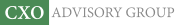Objective research to aid investing decisions

#### Value Investing Strategy (Strategy Overview)

Allocations for January 2021 (Final)
Cash TLT LQD SPY

#### Momentum Investing Strategy (Strategy Overview)

Allocations for January 2021 (Final)
1st ETF 2nd ETF 3rd ETF

# Timing the Dividend Risk Premium

| | Posted in: Equity Premium

Do stock dividends exhibit exploitable risk premiums? In their July 2018 paper entitled "A Model-Free Term Structure of U.S. Dividend Premiums", Maxim Ulrich, Stephan Florig and Christian Wuchte construct a term structure of the dividend risk premium and test strategies to time this premium at specific horizons. They specify dividend risk premium as the spread between:

• Expected dividend growth rate based on analyst 1-year and 2-year S&P 500 dividend forecasts, extended by analyst 5-year earnings growth estimates assuming constant future payout ratio.
• Expected dividend growth rate derived from equity index put and call option prices across different maturities.

They model an S&P 500 dividend capture portfolio for a given horizon as: long an S&P 500 Index put option of maturity matching the horizon; short an index call option of same maturity and strike price; long the index; and, short the money market in an amount matched to the option strike price. They test two strategies for capturing this premium at a 12-month horizon: (1) each month (last trading day) reform and hold the dividend capture portfolio; or, (2) each month reform and hold the dividend capture portfolio only when the dividend risk premium is positive (analyst-estimated dividends are higher than options-implied dividends). They model the risk-free rate/money market rate across horizons using the U.S. Dollar Overnight Index Swap rate for one day to 10 years. For the S&P 500 Index, they assume annual expense ratio 0.07% and 0.01% average bid-ask spread. For options, they estimate trading frictions with actual bid-ask spreads. Using S&P 500 Index/options and analyst forecast data as specified during January 2004 through October 2017, they find that: